## For a function g(x), the difference quotient is StartFraction 6 Superscript x + h minus 3 Baseline minus 6 Superscript x + 3 Baseline Over h

Question

For a function g(x), the difference quotient is StartFraction 6 Superscript x + h minus 3 Baseline minus 6 Superscript x + 3 Baseline Over h EndFraction. What is the average rate of change of g(x) on the interval from x = –2 to x = 1?

in progress 0
5 months 2021-08-30T09:35:47+00:00 1 Answers 0 views 0

## Answers ( )

The average rate of change in that interval is 1.99

Step-by-step explanation:

Here we have that the difference quotient for g(x) is: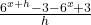Remember that for a general function f(x), the difference quotient is: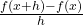So if we look at the difference quotient for g(x), we can conclude that: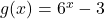Also remember that the average rate of change in an interval (a, b) is just: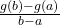So here we want the average rate of change of g(x) in the interval from x = -2 to x = 1, this is: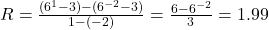The average rate of change in that interval is 1.99